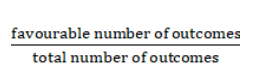# A die is thrown twice.

Question:

A die is thrown twice. What is the probability that at least one of the two throws comes up with the number 4?

Solution:

Given : A die is thrown twice

To find : Probability that at least one of the two throws comes up with the number 4

The formula used : Probability =A die is numbered from 1 to 6

When a die is thrown twice, total number of outcomes $=6^{2}=36$

Favourable outcomes =

$\{(4,1),(4,2),(4,3),(4,4),(4,5),(4,6),(1,4),(2,4),(3,4),(5,4),(6,4)\}$

Favourable number of outcomes = 11

Probability that at least one of the two throws comes up with the number $4=\frac{11}{36}$

The probability that at least one of the two throws comes up with the number $4=\frac{11}{36}$